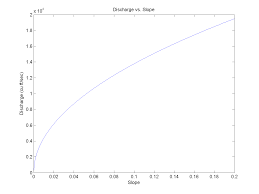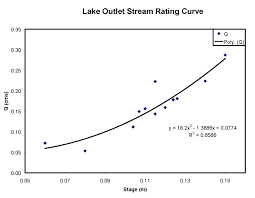## How to Calculate and Solve for Discharge | Chezy’s Equation | Water BudgetThe image above represents discharge | Chezy’s Equation.

To compute for discharge, four essential parameters are needed and these parameters are Area (A), Chezy’s Constant (C), Hydraulic Radius (R) and Slope (S).

The formula for calculating discharge:

Q = AC√(RS)

Where;

Q = Discharge
A = Area
C = Chezy’s Constant
S = Slope

Let’s solve an example;
Find the discharge when the area is 25, the chezy’s constant is 16, the hydraulic radius is 9 and the slope is 21.

This implies that;

A = Area = 25
C = Chezy’s Constant = 16
R = Hydraulic Radius = 9
S = Slope = 21

Q = AC√(RS)
Q = (25)(16)√((9)(21))
Q = (25)(16)√(189)
Q = (25)(16)(13.74)
Q = 5499.09

Therefore, the discharge according to Chezy’s equation is 5499.09.

Calculating the Area when the Discharge, the Chezy’s Constant, the Hydraulic Radius and the Slope is Given.

A = Q / C √RS

Where;

A = Area
Q = Discharge
C = Chezy’s Constant
S = Slope

Let’s solve an example;
Find the area when the discharge is 40, the chezy’s constant is 10, the hydraulic radius is 5 and the slope is 9.

This implies that;

Q = Discharge = 40
C = Chezy’s Constant = 10
R = Hydraulic Radius = 5
S = Slope = 9

A = Q / C √RS
A = 40 / 10 √(5)(9)
A = 40 / 10 √45
A = 40 / 10 (6.708)
A = 40 / 67.08
A = 0.596

Therefore, the area is 0.596.

## How to Calculate and Solve for Discharge | Slope Area Method | Water BudgetThe image above represents discharge | slope area method.

To compute for discharge | slope area method, four essential parameters are needed and these parameters are Manning’s Co-efficient (N), Area (A), Hydraulic Radius (R) and Slope (S).

The formula for calculating discharge | slope area method:

Q = (A / N) R2/3 S1/2

Where;

Q = Discharge
N = Manning’s Co-efficient
A = Area
S = Slope

Let’s solve an example;
Find the discharge when the manning’s co-efficient is 4, the area is 30, the hydraulic radius is 15 and the slope is 22.

This implies that;

N = Manning’s Co-efficient = 4
A = Area = 30
R = Hydraulic Radius = 15
S = Slope = 22

Q = (A / N) R2/3 S1/2
Q = (30 / 4) (15)2/3 (22)1/2
Q = (7.5) (6.08) (4.69)
Q = 213.96

Therefore, the discharge is 213.96.